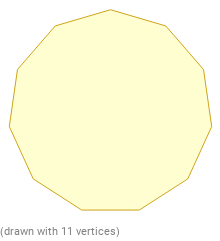Search IntMath
Close

450+ Math Lessons written by Math Professors and Teachers

5 Million+ Students Helped Each Year

1200+ Articles Written by Math Educators and Enthusiasts

Simplifying and Teaching Math for Over 23 Years

# Exterior Angles of a Polygon in Geometry## What are Polygons?

A polygon is a two-dimensional shape, with straight sides. There are three types of polygons: regular, irregular and convex. Regular polygons have equal sides and angles, while irregular polygons have unequal sides and angles. Convex polygons have all internal angles less than 180°.

## What are Polygon Angles?

The angles of a polygon are the angles between the sides. A regular polygon has equal angles and the sum of the internal angles is given by the formula 180(n-2), where n is the number of sides of the polygon. For example, the sum of the internal angles of a triangle is 180°, for a quadrilateral it is 360°, for a pentagon it is 540°, and for a hexagon it is 720°.

## What are Exterior Angles?

Exterior angles are the angles formed between one side of a polygon and the next. They are supplementary to the interior angles, meaning that the sum of an exterior angle and its adjacent interior angle is 180°. The sum of the exterior angles of a polygon is 360°.

## Finding the Measure of an Exterior Angle

The measure of an exterior angle of a polygon can be found by dividing the sum of the interior angles by the number of sides. For example, for a triangle, the sum of the interior angles is 180°, so the measure of an exterior angle is 180°/3 = 60°. For a quadrilateral, the sum of the interior angles is 360°, so the measure of an exterior angle is 360°/4 = 90°.

## Practice Problems

1. Find the sum of the exterior angles of a hexagon.

2. Find the measure of an exterior angle of a pentagon.

3. Find the measure of an exterior angle of a 7-sided polygon.

4. Find the measure of an exterior angle of an 11-sided polygon.

5. Find the sum of the interior angles of a 9-sided polygon.

6. Find the measure of an exterior angle of a 12-sided polygon.

7. Find the sum of the exterior angles of a 8-sided polygon.

## Summary

In this article, we discussed polygons, polygon angles, exterior angles and how to find the measure of an exterior angle. Exterior angles are the angles formed between one side of a polygon and the next and the sum of the exterior angles of a polygon is 360°. The measure of an exterior angle can be found by dividing the sum of the interior angles by the number of sides.

## FAQ

### What is an exterior angle of a polygon?

An exterior angle of a polygon is an angle outside of the polygon that is formed by a side of the polygon and the line extended from the next side.

### How many exterior angles does a polygon have?

A polygon has exactly as many exterior angles as it has sides.

## Problem SolverThis tool combines the power of mathematical computation engine that excels at solving mathematical formulas with the power of GPT large language models to parse and generate natural language. This creates math problem solver thats more accurate than ChatGPT, more flexible than a calculator, and faster answers than a human tutor. Learn More.